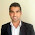2nd year paper scheme for chemistry 2022 is issued by all educational boards of Punjab to facilitate the students in their paper preparation. The scheme provides an understanding of the assessment pattern of the board for chemistry students. The students find it helpful in analyzing the importance and weightage of various sections of the course content.

# 2nd year chemistry paper scheme 2022Although I have give it the heading for the Lahore board, yet the scheme is for all Punjab boards. All the boards in Punjab follow this scheme.

This scheme for 12th class chemistry is for the Lahore board, Gujranwala board, Sargodha board, Multan Board, Rawalpindi board, Faisalabad board, DG Khan Board and Sahiwal board.

You may also like to see:
I have given here the detailed scheme in texts as well as an image of the scheme.

## 12th class chemistry pairing scheme 2022 all Punjab boards

The image form for the paper scheme is given below. You can download it in pdf too. Or copy the text of the pairing scheme to your phone. This is new paper scheme for FSc part 2 chemistry for 2022.

The FSc part 2 chemistry scheme is given below:

## HTML Table

Chapter No MCQs S.Qs L.Qs
1 1 2 1
2 1 2 1
3 1 2 1
4 1 2 NIL
5 1 3 NIL
6 1 2 1
7 1 2 1
8 1 3 1
9 1 2 1
10 1 2 1
11 1 2 1
12 1 1 1
13 1 2 NIL
14 1 2 NIL
15 1 2 May be
16 2 2 NIL

Q#2(Ch# 1, 2, 3, 4, 6, 15)       Q#3(Ch# 5, 7, 8, 10, 14)

Q#4(Ch# 9, 11, 12, 13, 16)     Q#5(Ch# 1, 3)      Q#6(Ch# 2, 6(or 15)

Q#7(Ch# 7, 10)       Q#8(Ch# 8, 12)       Q#9(Ch# 9, 11)

## 2nd year chemistry chapter wise long and short questions

Question No. 1 MCQs = 17 (1 mcqs from all chapters but 2 MCQs from chapter 12, 13, 15

Question No. 2 (8/12)
Chapter 1 = 2 short questions
Chapter 2 = 2 short questions
Chapter 3 = 2 short questions
Chapter 4 = 2 short questions
Chapter 6 = 2 short questions
Chapter 15 = 2 short questions

Question No. 3 (8/12)
Chapter 5 = 3 short questions
Chapter 7= 2 short questions
Chapter 8 = 3 short questions
Chapter 10 = 2 short questions
Chapter 14 = 2 short questions

Question No. 4 (6/9)
Chapter 9 = 2 short questions
Chapter 11 = 2 short questions
Chapter 12 = 1 short questions
Chapter 13 = 2 short questions
Chapter 16 = 2 short questions

Long questions (Attempt ANY THREE questions from FIVE Questions)

Questions No. 5
Chapter 1= part A
Chapter 3 = Part B

Questions No. 6
Chapter 2 = part A
Chapter 6 = Part B

Questions No. 7
Chapter 7 = part A
Chapter 10 = Part B

Questions No. 8
Chapter 8 = part A
Chapter 12 = Part B

Questions No. 9
Chapter 9 = part A
Chapter 11 = Part B

Relevant posts:
2nd year notes pdf
2nd year chemistry pairing scheme 2022Reviewed by Saif Ullah ZahidonMay 18, 2022Rating: 5

1.Sir chapter 16 is not included in reduce syllabus then why it is present in pairing scheme of chemistry for class 12 Sargodho board

1.Yes... Thank you.. but abhi this is old 2020.. I will update it on January 30, 2021.

2.Sir change to ni ho gi na

3.sir ap na update kr di ha ya nahi

4.Is this pairing scheme for 2022??

2.Waiting for 30 January ....

1.Updated.. new scheme for 2021 now given above.

2.Sir muje pairing scheme send kr dy f.sc ki

3.Sir thanks it is helpfull but one confusion I have that it is 100% true or not?

3.Sir plz upload chemistry scheme 2021

1.Updated dear.. now this is for 2021

2.Is it official..??

4.Sir ye official ha??

5.Sir long questions kee jo chapter 7 sa 12 tak scheem ha wo real hee ha

6.Bhot shukriya sir

7.sir i just need a 2nd yaer computer science chapter wise test seires

8.Sir chem ki scheme confirm hy ya copy pasye hy yh

9.Sir this is not matching with fsd pairing..Where 2 L.Q pairing is 4 and 6...And 3rd is chapter 7 and 12..But here pairing is different??

10.sir plz upload physis nume5rical pairing

11.Ye scheme chemistry ki change to Ni hogi nh??

12.Sir y offical hy

13.Sir ya Scheme confirm ha

14.Sir ye scheme bilkul perfect h smart syllabus k hisaab sy.?

15.Yeh real hai ya nhi

16.will long question appeared fromchapter 16

17.Sir muja mirpur board ki scheme chiye